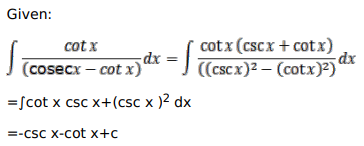# Mark against the correct answer in each of the following:

Question:

Mark $(\sqrt{)}$ against the correct answer in each of the following:

$\int \frac{\cot x}{(\operatorname{cosec} x-\cot x)} d x=?$

A. $=\operatorname{cosec} x-\cot x-x+C$

B. $\operatorname{cosec} x-\cot x-x+C$

C. $-\operatorname{cosec} x+\cot x-x+C$

D. $\operatorname{cosec} x+\cot x-x+C$

Solution: